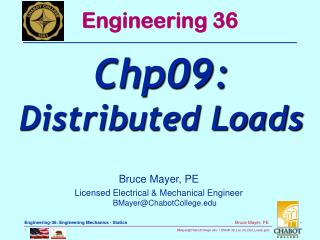Download PresentationBruce Mayer, PE Licensed Electrical & Mechanical Engineer BMayer@ChabotCollege# Bruce Mayer, PE Licensed Electrical & Mechanical Engineer BMayer@ChabotCollege - PowerPoint PPT Presentation

Download Presentation##### Bruce Mayer, PE Licensed Electrical & Mechanical Engineer BMayer@ChabotCollege

Download Policy: Content on the Website is provided to you AS IS for your information and personal use and may not be sold / licensed / shared on other websites without getting consent from its author. While downloading, if for some reason you are not able to download a presentation, the publisher may have deleted the file from their server.

- - - - - - - - - - - - - - - - - - - - - - - - - - - E N D - - - - - - - - - - - - - - - - - - - - - - - - - - -
##### Presentation Transcript

1. Engineering 36 Chp09: Distributed Loads Bruce Mayer, PE Licensed Electrical & Mechanical EngineerBMayer@ChabotCollege.edu

3. Distributed Loads • If the Load Profile, w(x), is known then the distributed load can be replaced with at POINT Load at a SPECIFIC Location • Magnitude of thePoint Load, W, is Determined by Area Under the Profile Curve

4. Distributed Loads • To Determine the Point Load Location employ Moments (1st Moment of Force) • Recall: Moment = [LeverArm]•[Intensity] • In This Case • LeverArm = The distance from the Baseline Origin, xn • Intensity = The Increment of Load, dWn, which is that load, w(xn) covering a distance dx located at xn • That is: dWn = w(xn)•dx

5. Distributed Loads • Now Use Centroidal Methodology • And Recall: • Equating the Ω Expressionsfind

6. A distributed load is represented by plotting the load per unit length, w (N/m). The total load is equal to the area under the load curve. • A distributed load can be REPLACED by a concentrated load with a magnitude equal to the area under the load curve and a line of action passing through the areal centroid. Distributed Loads on Beams

7. A beam supports a distributed load as shown. Determine the equivalent concentrated load and the reactions at the supports. Solution Plan The magnitude of the concentrated load is equal to the total load (the area under the curve) The line of action of the concentrated load passes through the centroid of the area under the Load curve. Determine the support reactions by summing moments about the beam ends Example:Trapezoidal Load Profile

8. Example:Trapezoidal Load Profile • SOLUTION: • The magnitude of the concentrated load is equal to the total load, or the area under the curve. • The line of action of the concentrated load passes through the area centroid of the curve.

9. Example:Trapezoidal Load Profile • Determine the support reactions by summing moments about the beam ends After Replacing the Dist-Load with the Equivalent POINT-Load Ay By

10. 3D Distributed Loads • The Previous 2D Dist Load Profile had units of Force per Unit-Length (e.g., lb/in or N/m) • If 3D The Force acts over an AREA and the units become Force per Unit Area, or PRESSURE (e.g., psi or Pa) • Knowledge of the Pressure Profile allows calculation of an Equivalent Point Load and its Location

11. Consider an Area Subject to a Pressure Load Uniform Pressure Profile Pressure Loading • The incremental Force, dFmn, Results from pressure p(xm,yn) acting on the incremental area dAmn= (dxm) (dyn) • Then the Total Force, F, on the Area

12. The Differential Geometry is shown below Pressure Loading: Total Force • Then the Total Pressure Force

13. Use MOMENT Methodology in 2-Dimensions to find the Location for the Point Force Fp Pressure Loading – Pressure Ctr • Then the Moment about the y-axis due to intensity dFmn and LeverArm xm • Then the Total y-axis Moment

14. Recall also Ωx = XC•Fp Pressure Loading – Pressure Ctr • Equating the two Ω expressions • The Similar Expression for YC • Isolating XC

15. Pressure Loading Summarized • Given a surface with Pressure Profile • The Equivalent Force, Fp, Exerted on the Surface due to the Pressure • Fp is located at the Center of Pressure at CoOrds (XC,YC)

16. WhiteBoard Work Lets WorkThese NiceProblems

17. Engineering 36 Appendix Bruce Mayer, PE Registered Electrical & Mechanical EngineerBMayer@ChabotCollege.edu

18. Beam Problem • For the Negligible-Wt Beam Find • Equivalent POINT-Load and it’s Location (Point of Application, PoA) • The RCNs at Pt-A

19. Pressure Problem • Find the Equivalent POINT-LOAD and its Point of Application (Location) For the Given Pressure Distribution

20. The Differential Geometry is shown belwo Pressure Loading • Then the Total Pressure Force